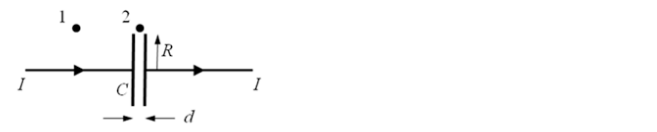# Problem: A constant current I is supplied for a brief time to charge a parallel plate capacitor. The capacitor has circular plates of radius R with gap d (d &lt;&lt; R). Point 1 is at R + d from the wire, and point 2, is at a distance R + d from the center of the capacitor. After the capacitor is charged and the current I goes to zero a) the electric flux and the magnetic field between the plates are both zero. b) the electric flux between the plates is zero and magnetic field between the plates is non-zero. c) the electric flux between the plates is non-zero and the magnetic field between the plates is zero.

🤓 Based on our data, we think this question is relevant for Professor Dawson's class at UTAH.

###### Problem Details

A constant current I is supplied for a brief time to charge a parallel plate capacitor. The capacitor has circular plates of radius R with gap d (d << R). Point 1 is at R + d from the wire, and point 2, is at a distance R + d from the center of the capacitor.

After the capacitor is charged and the current I goes to zero

a) the electric flux and the magnetic field between the plates are both zero.

b) the electric flux between the plates is zero and magnetic field between the plates is non-zero.

c) the electric flux between the plates is non-zero and the magnetic field between the plates is zero.